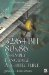Exercises1. Using only Boolean logic, how could two numbers be summed?

2. If your processor had no instructions for parallel subtraction, how would you find the difference of two numbers?

3. Invert the sign of the even-numbered elements of signed 8-bit byte, 16-bit half-word, and 32-bit word of a 128-bit data value using:

1. pseudo vector C code

2. MMX

3. SSE2

4. Same as problem 3 but use odd-numbered elements.

5. Invert the sign of all the elements of four packed single-precision floating-point values.

6. You have been given a 4096-byte audio sample consisting of left and right channel components with a PCM (pulse coded modulation) of unsigned 16-bit with 0x8000 as the baseline.

unsigned short leftStereo, rightStereo;  signed char Mono[???];
7. How many bytes is the mixed sample?

8. Write a mixer function to sum the two channels from stereo into mono and convert to a signed 8-bit sample.

Project:

You now have enough information to write an SHA-1 algorithm discussed in Chapter 5, "Bit Wrangling," for your favorite processor. Write one! HINT: Write the function code in C first.32/64-Bit 80x86 Assembly Language Architecture
ISBN: 1598220020
EAN: 2147483647
Year: 2003
Pages: 191

Similar book on Amazon